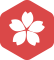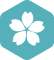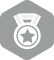### 1+1=22014年10月 扩充话题大版内专家分月排行榜第三GitHub 绑定GitHub第三方账户获取2014年11月 Linux/Unix社区大版内专家分月排行榜第一
2014年10月 Linux/Unix社区大版内专家分月排行榜第一
2014年9月 Linux/Unix社区大版内专家分月排行榜第一
2014年8月 Linux/Unix社区大版内专家分月排行榜第一2003年2月 C/C++大版内专家分月排行榜第三2014年11月论坛优秀版主2017年7月 扩充话题大版内专家分月排行榜第一
2017年6月 扩充话题大版内专家分月排行榜第一
2017年5月 扩充话题大版内专家分月排行榜第一
2017年3月 扩充话题大版内专家分月排行榜第一
2017年1月 扩充话题大版内专家分月排行榜第一
2016年12月 扩充话题大版内专家分月排行榜第一
2016年11月 扩充话题大版内专家分月排行榜第一
2016年10月 扩充话题大版内专家分月排行榜第一
2014年2月 扩充话题大版内专家分月排行榜第一
2014年1月 扩充话题大版内专家分月排行榜第一2018年2月 扩充话题大版内专家分月排行榜第二
2017年9月 扩充话题大版内专家分月排行榜第二
2017年2月 扩充话题大版内专家分月排行榜第二1+1=2的 blog 文章索引

1+1=2   好吧,我承认这是小学生也会做的题目,但是作为一个程序员,或者是软件设计师,你知道1+1=2如何在计算机内部表示并执行的么?  在复习软件设计师中的计算机组成原理的时候这个问题一直在...

1+1/2-1/3+.......+1/100(C)

#include&lt;stdio.h&gt; int main() { int sign=1; double fm=2.0,sum=1.0,term; while(fm&lt;=100) { sign=-sign; term=sign/fm; sum=sum+term; fm=f...

cmake 学习笔记(一)

s1=s1+1;有什么错？ * short s2=1;s2+=1;有什么错？ * long l1 = 1;l1 = l1+1;有什么错？ * long l2 = 1;l2+=1;有什么错？ * 对于short s1 = 1; s1 = s1 + 1; 由于s1+1运算时会自动提升表达式的类型，所以结果是...

C语言：编写函数fun，...n通过形参传入，S=1+1/(1+2)+1/(1+2+3)+……1/(1+2+3+…+n) 例如：若n的值为11，函数

#include<conio.h> #include<stdio.h> #include<string.h>...S=1+1/(1+2)+1/(1+2+3)+……1/(1+2+3+…+n) 例如：若n的值为11时，函数的值为1.833333。 注意：部分源程序给出如...

C语言：编写一个程序，求s=1+(1+2)+(1+2+3)+....+(1+2+3+....+n)的值。

/*编写一个程序，求s=1+(1+2)+(1+2+3)+....+(1+2+3+....+n)的值*/ #include <stdio.h> int main() { int i,n,sum=0,result=0; printf("input n:\n"); scanf("%d",&n); for(i=1;i<=n;i++) { sum+=...

C语言：求1+1/2+1/4+1/7+1/11+1/16+1/22+…的值，直到最后一项的值小于10的-5次幂

1+1/2+1/4+1/7+1/11+1/16+1/22+…的值，直到最后一项的值小于10^-5。 其中a_n=1/(n+1/a_n-1)， a_0=1， n=1,2,3… 注意事项 总和的最后一项是小于10^-5的，因此在代码中我在循坏体外多写了一次。 为方便理解，...

Java实现 蓝桥杯 历届真题 数字拆分

/* * Copyright (c) 2011, 烟台大学计算机学院 * All rights reserved. * 文件名称：test.cpp * 作 者：樊露露 * 完成日期：2012 年 10 月 29 日 ...* 问题描述：计算并输出1+1/2+1/3+.

#include using namespace std; int main() { int n, sum; cout 请输入N：" ;...for (int i = 1; i ; i++) { for (int j = 1; j ; j++) { sum = sum + j; } } cout 结果为：" ; system("pause"); return 0; }

Java 输入n,打印1!+2!+3!+...+n!的值"-----用递归求解

import java.util.Scanner; class T23  { public static void main(String[] args)...System.out.println("输入n,打印1!+2!+3!+...+n!的值"); Scanner in=new Scanner(System.in); int n=in.nextInt(); while(n

1+1/2+1/3+1/4+...+1/n

#include <stdio.h>int main() { double sum=0; int n,i; scanf("%d",&n); printf("\n"); for(i=1;i;i++) sum=sum+1.0/i; printf("sum=%1f",sum); return 0; }运行结果：